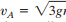### Create an Account

Home / Questions / The ball of mass m is given a speed of at position A When it reaches B the cord hits the...

# The ball of mass m is given a speed of at position A When it reaches B the cord hits the small peg P, after which the ball describes a smaller circular path Determine the position x of P so that

The ball of mass m is given a speed ofat position A. When it reaches B, the cord hits the small peg P, after which the ball describes a smaller circular path. Determine the position x of P so that the ball will just be able to reach point C.

Jun 03 2020 View more View LessSubscribe To Get Solution